## Solve Exercises Lesson 2: Equal triangles (C8 Math 7 Horizons) – Math Book

Solution for Exercise 2: Congruent triangles (C8 Math 7 Horizons)
========
==========

### Solution 1 page 57 Math textbook 7 Creative horizon volume 2 – CTST

Observe Figure 23 and then change the sign ? with the appropriate triangle name.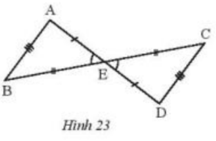a) △ABE = △ ?

b) △EAB = △?

c) ? =△CDE.

Detailed instructions for solving Lesson 1

Solution method

Use 3 equal cases of triangles.

Detailed explanation

Looking at Figure 23, we see:

a) Consider △ABE and △DCE having:

AB = DC (assumption).

BE = CE (assumption).

AE = DE (assumption).

It follows that ABE = DCE (ccc).

So ABE = DCE.

b) Do ABE = △DCE (proven above) so △EAB = △EDC.

c) Do ABE = DCE (proven above) so △BAE = CDE.

### Solve problem 2 page 57 Math textbook 7 Creative horizon volume 2 – CTST

Given \(\Delta{DEF}=\Delta{HIK}\) and \(\widehat D= {73^o}\), DE = 5cm, IK = 7cm. Calculate the measure \(\widehat H\) and the lengths HI, EF.

Detailed instructions for solving Lesson 2

Solution method

If two triangles are congruent, then their corresponding sides are congruent and their corresponding angles are congruent.

Detailed explanation

Because \(\Delta{DEF}=\Delta{HIK}\)

\( \Rightarrow \widehat D = \widehat H\)( 2 corresponding angles )

Which \(\widehat D =73^0\)

\( \Rightarrow \widehat H=73^0\)

Because \(\Delta{DEF}=\Delta{HIK}\)

\(\Rightarrow DE = HI;EF = IK;DF = HK\)( corresponding edges )

So \( \widehat H = {73^o};HI = 5cm;EF = 7cm\)

–>

— *****

### Solve problem 3 page 58 Math textbook 7 Creative horizon volume 2 – CTST

Given two congruent triangles ABC and DEF (unwritten vertices respectively), where \(\widehat A = \widehat E\), \(\widehat C = \widehat D\). Find pairs of equal sides, remaining pairs of corresponding angles.

Detailed instructions for solving Lesson 3

Solution method

The corresponding vertices angles are congruent, so the order of the vertices of the two triangles is congruent.

If two triangles are congruent, then the corresponding sides are congruent and the corresponding angles are congruent.

Detailed explanation

Since \(\widehat A = \widehat E\), \(\widehat C = \widehat D\) vertex A corresponds to vertex E, vertex C corresponds to vertex D.

\( \Rightarrow \widehat B = \widehat F\) ( 2 corresponding angles)

Therefore, \(\Delta{ABC}=\Delta{EFD}\)

\(\Rightarrow AB = DE;BC = EF;AC = DF\)( corresponding sides )

### Solve problem 4 page 58 Math textbook 7 Creative horizon volume 2 – CTST

Given that \(\Delta{MNP}=\Delta{DEF}\) and MN = 4cm, MP = 5cm, EF = 6cm. Calculate the perimeter of triangle MNP.

Detailed instructions for solving Lesson 4

Solution method

Use the product of the corresponding angles and sides of two triangles that are congruent

Detailed explanation

Because \(\Delta{MNP}=\Delta{DEF}\)

\( \Rightarrow DE = MN;EF = NP;DF = MP\) (corresponding edges)

\( \Rightarrow NP = 6cm\)

\( \Rightarrow \) The perimeter of triangle MNP is:

C = MN + MP + NP = 4 + 5 + 6 = 15 (cm)

–>

— *****

### Solve problem 5 page 58 Math textbook 7 Creative horizon volume 2 – CTST

Let AB be the line segment with O as the midpoint. Draw two lines m and n perpendicular to AB at A and B respectively. Taking the point C on m, CO intersects n at D (Figure 24). Prove that O is the midpoint of CD.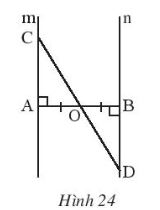Detailed instructions for solving Lesson 5

Solution method

– Prove that triangle OAC and triangle OBD are congruent

– From that deduce OC = OD (2 corresponding edges)

Detailed explanation

Considering \(\Delta OAC\) and \(\Delta OBD\), we have:

\(\widehat {COA} = \widehat {BOD}\) ( 2 opposite angles)

AO = BO

\(\widehat A = \widehat B\)

\(\Rightarrow \Delta OAC=\Delta OBD\) ( gcg )

\( \Rightarrow CO = DO\) (corresponding edge )

\( \Rightarrow \) O is the midpoint of CD

–>

— *****

### Solve problem 6 page 58 Math textbook 7 Creative horizon volume 2 – CTST

Given Figure 25 with EF = HG, EG = HF. Prove that:

a) \(\Delta EFH=\Delta HGE\)

b) EF // HG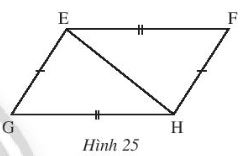Detailed instructions for solving Lesson 6

Solution method

– Prove that 2 triangles are congruent (ccc)

– Prove that 2 angles in staggered positions are congruent

Detailed explanation

a) Consider triangle EGH and triangle HFE with :

FE = GH; GE = HF; General EH

\(\Rightarrow \Delta EFH=\Delta HGE\) (ccc)

\( \Rightarrow \widehat {FEH} = \widehat {EHG}\)( 2 corresponding corners )

b) Because \(\widehat {FEH}=\widehat {EHG}\)

But these 2 angles are in staggered position in

Therefore, EF // HG

–>

— *****

### Solve problem 7 page 58 Math textbook 7 Creative horizon volume 2 – CTST

Let the triangle FGH have FG = FH. Take a point I on the side GH such that FI is the bisector of \(\widehat {GFH}\).Prove that the two triangles FIG and FIH are congruent.

Detailed explanation guide

Solution method

2 triangles are congruent according to the case cgc

Detailed explanation

Consider triangles FIG and FIH with:

General FI

FG = FH (assumption)

\(\widehat {GFI} = \widehat {HFI}\) (since FI is the bisector \(\widehat {GFH}\))

\(\Rightarrow \Delta FIG=\Delta FIH\) (cgc)

–>

— *****

### Solve problem 8 page 58 Math textbook 7 Creative horizon volume 2 – CTST

Given the angle xOy. Take two points A and B on the ray Ox such that OA < OB. Take two points C and D on the ray Oy such that OC = OA, OD = OB. Let E be the intersection of AD and BC. Prove that:

b) \(\Delta EAB=\Delta ECD\)

c) OE is the bisector of angle xOy.

Detailed instructions for solving Lesson 8

Solution method

Using equal cases of triangles: ccc; cgc; gcg to prove the triangles are congruent and then deduce the corresponding sides are equal or the corresponding angles are congruent

Detailed explanation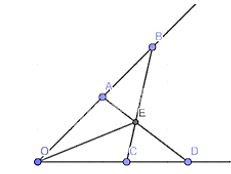a) Considering \(\Delta OAD\) and \(\Delta OCB\), we have :

OD = OB

\(\widehat{A}\) general

OA = OC

\(\Rightarrow \Delta OAD=\Delta OCB\) (cgc )

\( \Rightarrow AD = BC\)(2 corresponding edges )

\(\widehat{OCB}+\widehat{BCD}=180^0\) ( 2 complementary angles)

Because \(OA+AB=OB; OC+CD=OD\)

Where \(OC = OA, OD = OB\)

\(\Rightarrow AB=CD\)

Considering \(\Delta EAB\) and \(\Delta ECD\), we have:

\(\widehat {ABE} = \widehat {CDE}\)

\(AB = CD\)

\(\widehat {BAE} = \widehat {DCE}\)

\(\Rightarrow \Delta EAB=\Delta ECD\) (gcg)

c) Since \(\Delta EAB=\Delta ECD\) EB = ED ( 2 corresponding edges)

Considering \(\Delta OBE\) and \(\Delta ODE\), we have :

EB = ED

OB = OD

General OE

\( \Rightarrow \Delta OBE=\Delta ODE \) (ccc)

\( \Rightarrow \widehat{BOE}=\widehat{DOE}\) ( 2 corresponding corners)

\( \Rightarrow \) OE is the bisector \(\widehat {xOy}\)

–>

— *****

### Solve problem 9 page 58 Math textbook 7 Creative horizon volume 2 – CTST

Name some of the points in Figure 26 and indicate three pairs of congruent triangles in the figure.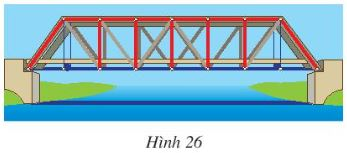Detailed instructions for solving Lesson 9

Solution method

We name and then rely on the equal line segments to find pairs of similar triangles

Detailed explanation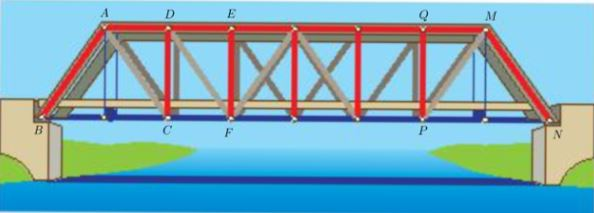Name the points as shown above.

Based on the above figure, we have the following pairs of congruent triangles:

△ABC = △MNP;